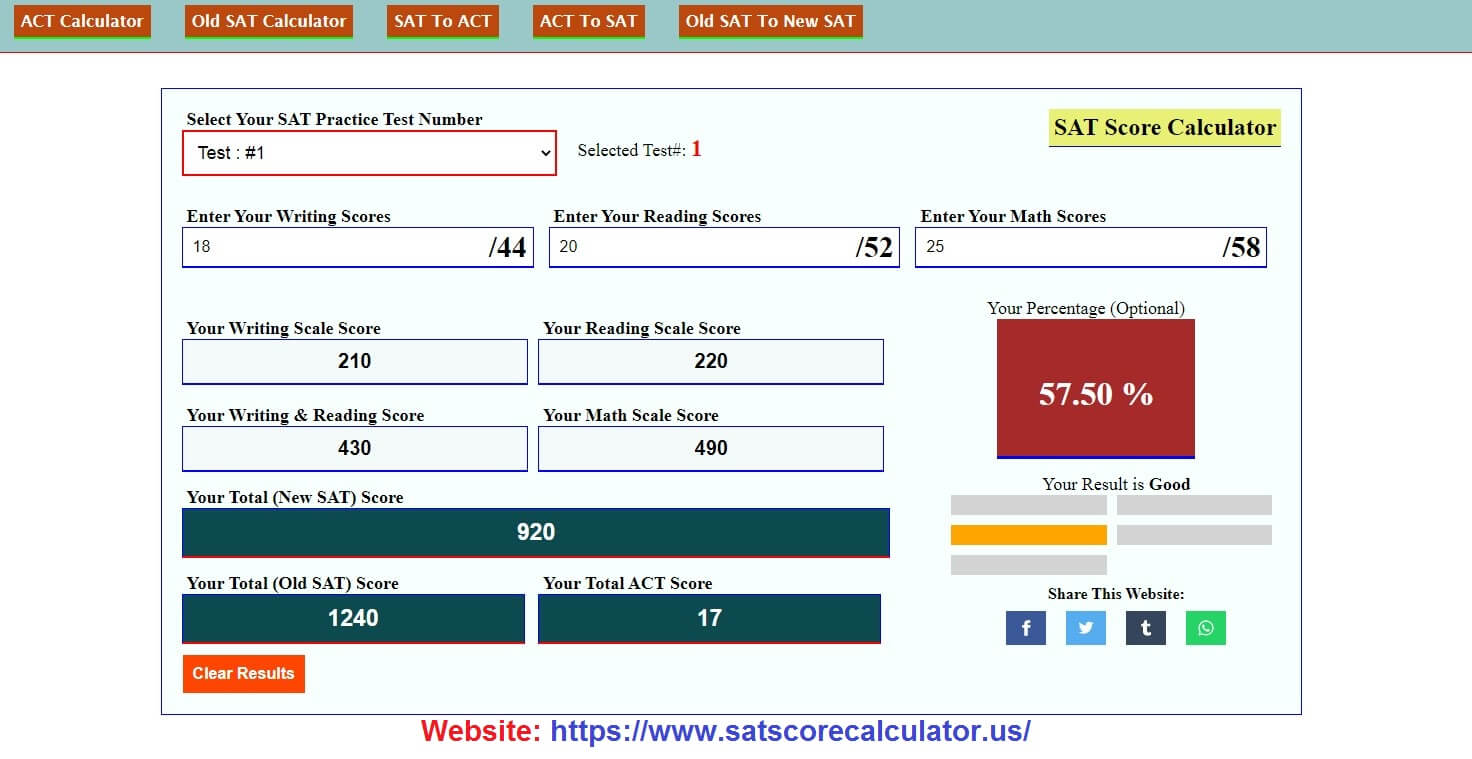# SAT Score Calculator

Selected Test#:

## SAT Calculator

It is an online calculation tool 2022, where you can easily calculate your SAT Score. First, you can select a sat test we have added 10 sat practice tests. Sat Practice Test 1, Sat Practice Test 2, Sat Practice Test 3, Sat Practice Test 4, Sat Practice Test 5, Sat Practice Test 6, Sat Practice Test 7, Sat Practice Test 8, Sat Practice Test 9, Sat Practice Test 10. After that Just enter your writing score, reading score, and Math score. and on the basis of results, you can take the decision to go to school and college. On this page, we have done the calculation of SAT, and with the same data or Input scores, you can get New SAT results, Old SAT, and more in one place, Also we have done your score percentage option where you can easily know that in which percentage you got your score.

### What is SAT?

The Scholastic Aptitude Test is a standardized test used for college admissions in the United States. Its Score And Name Changed Multiple Times. Later Again it is called the Scholastic Assessment Test, The SAT is developed and owned by Colleges Board in the United States.

#### How To Calculate SAT Score?

At first, Select Practice Test Number: by default, it is Test 1
Enter Score in Writing Textbox: Max(44)
Enter Score in Reading Textbox: Max(52)
Enter Score in Math Textbox: Max(58)
For the given Test Number and Scores you will get results for SAT, Old SAT, ACT, Optional Percentage, and Optional Status.
Optional Percentage is calculated for your SAT Score from Total SAT Score and Optional Status is calculated on the Percentage base. 0% to 20% = Low
20% to 40% = Average
40% to 60% = Good
60% to 80% = Best
80% to 100% = Excellent

Formula : (Reading Scale Score + Writing Scale Score) + Math Scale Score = Total SAT Score
exp: (210 + 220) + 490 = 920 Total SAT Score

##### Highest Sat score/Lowest Sat Score/Sat Score Max/Perfect Sat Score

The highest SAT score you can get is 1600. And The lowest SAT score is 400. And The Perfect SAT Score Will Be To Get At Least (1150-1200). Come To Calculate It Now.

###### Sat Scores Range

SAT total scores range from (400 to 1600). the highest is (1600) and the lowest is (400). Now It depends on you how many high and low scores you can get.

What Is a good Sat Calculator Score? What Is An Average Sat score?

The Good SAT score range is 1100 to 1200 to go into good schools and colleges. College Board Selection is under 1150 is an average sat score. And if you get above 1150 then it will be considered above the national average score. Calculate it now.No posts.
No posts.